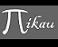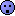Discussion about math, puzzles, games and fun.   Useful symbols: ÷ × ½ √ ∞ ≠ ≤ ≥ ≈ ⇒ ± ∈ Δ θ ∴ ∑ ∫  π  -¹ ² ³ °

You are not logged in.

## #1 2007-12-04 06:17:54

mikau
MemberRegistered: 2005-08-22
Posts: 1,504

### Matrix multiplication and inner products

I had a weird thought the other night as i was dozing off.

the product of two matrices A and B  (AB) is the matrix who's (i,j)th element is the dot product of the i'th row of matrix A and the j'th collumn of matrix B.

What if we were to redefine matrix multplication such that it is always associated with some inner product of two vectors in R^n. That is, suppose we define a place where the (i,j)th element of AB is equal to the inner product P of the i'th row of A and the j'th collumn of B.

Then the whole of study of matrices (at least as far as I know it) is reduced to a mere subset of all possible matrix laws in which the inner product is chosen to be the dot product.and now of course i'd like to know, has this been thought of already?A logarithm is just a misspelled algorithm.

Offline

## #2 2019-05-13 11:22:31

Alg Num Theory
MemberRegistered: 2017-11-24
Posts: 693
Website

### Re: Matrix multiplication and inner products

Dot product is matrix multiplication. The dot product of the vectors

[list=*]
[*]

[/*]
[/list]

is the multiplication of the 1×n matrix

[list=*]
[*]

[/*]
[/list]

by the n×1 matrix

[list=*]
[*]

.[/*]
[/list]

Multiplication of matrices with more rows and columns is a way of doing multiple dot products simultaneously.

Me, or the ugly man, whatever (3,3,6)

Offline